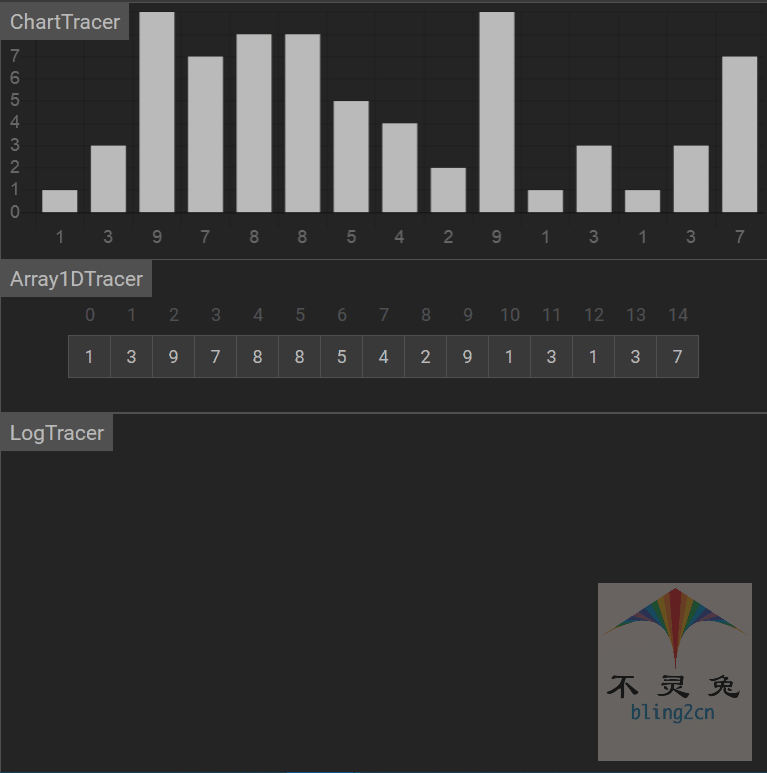# python实现•十大排序算法之快速排序(Quick Sort)

## 算法实现步骤

1. 首先设定一个分界值(pivot)，通过该分界值将数组分成左右两部分。
2. 将大于或等于分界值的数据集中到数组右边，小于分界值的数据集中到数组的左边。此时，左边部分中各元素都小于或等于分界值，而右边部分中各元素都大于或等于分界值,这个称为分区（partition）操作。
3. 然后，左边和右边的数据可以独立排序。对于左侧的数组数据，又可以取一个分界值，将该部分数据分成左右两部分，同样在左边放置较小值，右边放置较大值。右侧的数组数据也可以做类似处理。
4. 重复上述过程，通过递归（recursive）将左侧部分排好序后，再递归排好右侧部分的顺序。当左、右两个部分各数据排序完成后，整个数组的排序也就完成了。

## Python 代码实现

# quick_sort 代码实现

def partition(arr: List[int], low: int, high: int):
pivot, j = arr[low], low
for i in range(low + 1, high + 1):
if arr[i] <= pivot:
j += 1
arr[j], arr[i] = arr[i], arr[j]
arr[low], arr[j] = arr[j], arr[low]
return j

def quick_sort_between(arr: List[int], low: int, high: int):
if high-low <= 1: # 递归结束条件
return

m = partition(arr, low, high)  # arr[m] 作为划分标准
quick_sort_between(arr, low, m - 1)
quick_sort_between(arr, m + 1, high)

def quick_sort(arr:List[int]):
"""
快速排序(in-place)
:param arr: 待排序的List
:return: 快速排序是就地排序(in-place)
"""
quick_sort_between(arr,0, len(arr) - 1)

# 测试数据

if __name__ == '__main__':
import random
random.seed(54)
arr = [random.randint(0,100) for _ in range(10)]
print("原始数据：", arr)
quick_sort(arr)
print("快速排序结果：", arr)

# 输出结果



## 动画演示## 算法分析

• 时间复杂度

快速排序最优的情况就是每一次取到的元素都刚好平分整个数组，此时的时间复杂度公式则为：

$T\left[ n \right] =2T\left[ \dfrac{n}{2} \right] \ +\ f\left( n \right)$

$T\left[ n \right]$为平分后的子数组的时间复杂度，$f\left( n \right)$为平分这个数组时所花的时间；

则有：

\begin{align} T\left[ n \right] &=2T\left[ \dfrac{n}{2} \right] +n &\text{第1次递归}n=n\\ &=2^2T\left[ \dfrac{n}{2^2} \right] +2n &\text{第2次递归}n=\dfrac{n}{2}\\ & =2^3T\left[ \dfrac{n}{2^3} \right] +3n &\text{第3次递归}n=\dfrac{n}{2^2}\\ &\cdots \cdots \\ &=2^mT\left[ \dfrac{n}{2^m} \right] +mn &\text{第m次递归}n=\dfrac{n}{2^m} \end{align}

当最后平分的不能再平分时有：

\begin{align} &T\left[ \dfrac{n}{2^m} \right] =T\left[ 1 \right] \\ \Rightarrow &\frac{n}{2^m}=1 \\ \Rightarrow &m=\log _2n \\ \Rightarrow &T\left[ n \right] =n+n\log _2n=O(nlog_2n) \end{align}

快速排序最优的情况下时间复杂度为：$O\left( n\log _2n \right)$

最差的情况就是每一次取到的元素就是数组中最小/最大值，这种情况其实就是冒泡排序了(每一次都排好一个元素的顺序)，这种情况时间复杂度就是冒泡排序的时间复杂度：

$T\left[ n \right] =n\left( n-1 \right) =n^2+n=O\left( n^2 \right)$

快速排序最差的情况下时间复杂度为：$O(n^2)$

快速排序的平均时间复杂度也是：$O\left( n\log _2n \right)$

• 空间复杂度

首先就地快速排序使用的空间是$O(1)$的，也就是个常数级。真正消耗空间的就是递归调用了，因为每次递归就要保持一些数据。
最优的情况是每一次都平分数组，空间复杂度为：$O(log_2n)$
最差的情况是退化为冒泡排序，空间复杂度为：$O(n)$

• 稳定性

排序过程中，相同的元素无法保证相对位置不变，因此快速排序属于不稳定排序。

• 综合分析

时间复杂度(平均) 时间复杂度(最好) 时间复杂度(最坏) 空间复杂度 排序方式 稳定性
$O(nlog_2n)$ $O(nlog_2n)$ $O(n^2)$ $O(log_2n)$ in-place 不稳定

## 联系我们posted @ 2020-05-23 09:07  南风以南  阅读(48)  评论(0编辑  收藏  举报